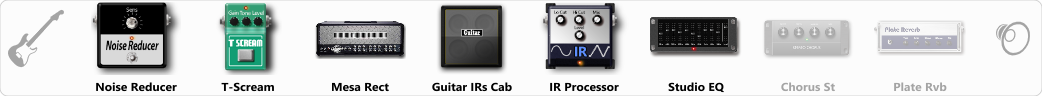# Yep

Discussion in 'ToneLib-GFX presets' started by edwincrain, Jan 3, 2020.

1. Yep

Preset name: Edwin's Triple Rectifire

Just goofing with eq and Ir's.

Effects chain:Effect: "Noise Reducer" (Dynamics / Filter), active - "yes"
"Sens" = 30
"Mode" = Soft

Effect: "T-Scream" (Overdrive / Distortion), active - "yes"
"Drive" = 9
"Tone" = 70
"Level" = 100

Effect: "Mesa Rect" (Amp simulators), active - "yes"
"Gain" = 52
"Bass" = 75
"Middle" = 52
"Treble" = 47
"Presence" = 68
"Master" = 59
"Output" = 50
"Level (dB)" = 1

Effect: "Guitar IRs Cab" (Cabinets), active - "yes"
"Model" = Mesa Rectifier (4x12")
"Mic Position" = Center
"Mic Distance" = Near
"Low Cut (Hz)" = 60
"Hi Cut (kHz)" = 20.0
"Mix" = 100
"Level (dB)" = 0

Effect: "IR Processor" (Dynamics / Filter), active - "yes"
"IR" = Recto112-V30-API_MD421-1
"Low Cut (Hz)" = 101
"Hi Cut (kHz)" = 9.5
"Mix" = 40
"Level (dB)" = 1

Effect: "Studio EQ" (Dynamics / Filter), active - "yes"
"31 Hz" = -15
"62 Hz" = -8
"125 Hz" = 3
"250 Hz" = -2
"500 Hz" = 2
"1 kHz" = 3
"2 kHz" = 6
"4 kHz" = -4
"8 kHz" = 4
"16 kHz" = 4
"above 16 kHz" = 0
"Level (dB)" = 6

Effect: "Chorus St" (Modulation / Sfx), active - "no"
"Speed" = 1.9
"Depth" = 21
"Center" = 2.9
"Mix" = 18

Effect: "Plate Rvb" (Reverb), active - "no"
"Time" = 4.3
"PreDelay" = 32
"LoDamp" = 22
"HiDamp" = 32
"Mix" = 46

Note: You will need to download and install the ToneLib-GFX software to use the preset.

#### Attached Files:

• ###### Yep.tlgfx
File size:
2.6 KB
Views:
5,822
Gilly, Azat and Arcanum like this.
2. It's good for St.Anger album!

edwincrain likes this.